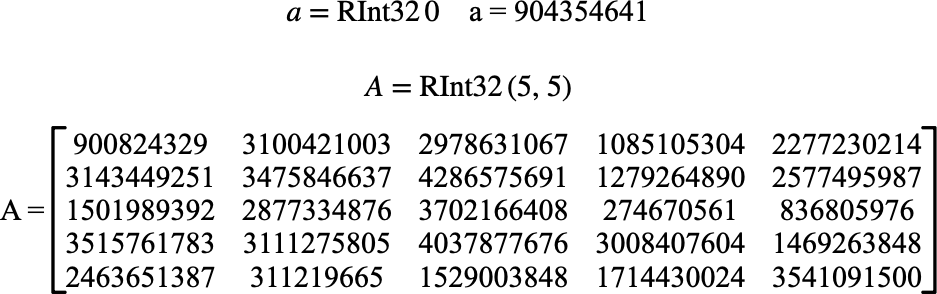# $$\text{RInt32}$$¶

You can use the $$RInt32$$ function to obtain a random 32-bit integer or a matrix of random 32-bit integers.

You can use the \rint32 backslash command to insert this function.

The following variants of this function are available:

• $$\text{integer } \text{RInt32} \left ( \text{<any value>} \right )$$

• $$\text{integer matrix } \text{RInt32} \left ( \text{<number rows}, \text{<number columns} \right )$$

The single value variant of the $$\text{RInt32}$$ function requires an arbitrary parameter value that is ignored.

The matrix variant of the $$\text{RInt32}$$ function requires two values indicating the desired matrix dimensions.

The returned integer value(s) are generated using the currently selected random number generator. The returned value(s) will be uniformly distributed over the range [0, 4294967295].

Figure 194 shows the basic use of the $$\text{RInt32}$$ function.Figure 194 Example Use Of The RInt32 Function